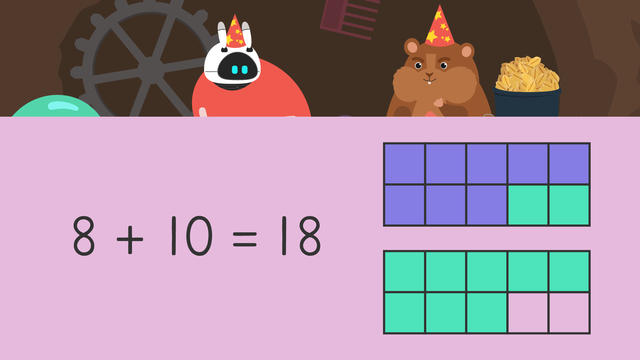Rating

Ø 5.0 / 1 ratings

The authorsTeam Digital
CCSS.MATH.CONTENT.2.OA.A.1

## Basics on the topicOrder in Adding

### Content

In this video Mr. Squeaks and Imani are getting ready for a birthday party! There is just one problem though, they can’t decide on the order of items they will add to the goody bags they will give their guests! Mr. Squeaks wants to add mini-erasers first, but Imani wants to add sprinkles first. Learn more about addition property of order below to see how they will solve this tricky problem.

#### Order of Numbers in Addition

What IS order in adding? When we talk about order in adding we're discussing which number to add first in an addition equation. This is also known as the commutative property or, the commutative order property of addition.

What is the commutative order property of addition? You may have heard grown ups say that cars commute, well did you know that numbers do too? Commute is another way to say travel. Cars travel from one place to another, and numbers can too!

When addends travel, or commute, in an equation, it means those numbers have moved, or, rearranged. Even when you rearrange the addends, the SUM, or total, will always be the same. When rearranging, or moving addends…

• Start by setting up an equation.
• Next, solve using a strategy that works for you, in this video we used ten frames.
• Once you have solved the first equation, create another by moving, or, rearranging the addends.
• Then, solve again using ten frames.

Even though the addends commuted, the sum remains the same!

#### Order of Operations Addition Example

Let’s practice order in adding with the example below.Mr. Squeaks wants to start by adding twenty-two seeds, but Imani wants to start with twelve flowers. How can we use order in adding to help them see their sum?

• Start by setting up an equation.
• Next, solve using a strategy that works for you, in this video we used ten frames.
• Once you have solved the first equation, create another by moving, or, rearranging the addends.
• Then, solve again using ten frames.What do you notice? The sum is the same!

#### Order in Adding - Summary

Today we learned about order in adding. That's when you decide which addend to add first. We also learned about the commutative property and commuting numbers. Remember, when addends commute, those numbers rearrange, but the sum ALWAYS stays the same...

#### Equal and Not Equal – Additional Practice

Want more practice? Take a look at the end of this video for changing order of addition property worksheets and exercises.

Would you like to apply the knowledge you’ve learned? You can review and practice it with the tasks for the video Order in Adding.
• ### Which equations does this show?

Hints

How many pencils and how many erasers were put in to each bag? These are your addends.

Solution

There are 7 pencils and 8 erasers in each bag.

7 + 8 = 15 and 8 + 7 = 15. These answers are both correct.

When we have two amounts to add together, we can add them in any order.

• ### Match the pairs that are equal equations.

Hints

What is the total of the first pair? First find the amount of green squares, then the amount of purple squares.

Is there another ten frame that has the same total but the addends are in the opposite order?

Solution

The image shows the pair that shows 5 + 8 = 13 and 8 + 5 = 13

----------------------------------------------

The other pairs are:

8 + 10 = 18 and 10 + 8 = 18

12 + 22 = 34 and 22 + 12 = 34

13 + 25 = 38 and 25 + 13 = 38

• ### Complete the equations.

Hints

The first number sentence is to add the purple and green, so count how many purple squares and put this number in the first gap.

The second number sentence is to add green and purple, so count how many green squares and put this number in the first gap.

The commutative property tells us that we can add the two addends in any order and we still get the same answer. For example, 4 + 6 = 10 and 6 + 4 = 10.

Solution

There are 14 purple and 18 green squares. These can be added together in both ways and the answer is still 32.

14 + 18 = 32 and 18 + 14 = 32.

• ### Which images answer the question?

Hints

There are two correct options.

Which tens frames have 16 and 8 shown in purple and green?

The total number of colored squares is equal to 16 + 8.

Solution
• There were two options that correctly displayed this problem.
• Since there were 16 balloons and 8 pieces of candy, we could do: 16 + 8 = 24 OR 8 + 16 = 24.
• ### How many treats are in each party bag?

Hints

Count how many heart stickers there are and how many star stickers there are then add these together.

Count how many stickers there are in total on the tens frames.

Solution
• There are 8 star stickers and 9 heart stickers.
• In total there are 17 stickers.
• 8 + 9 = 17
• 9 + 8 = 17
• ### Matching equations.

Hints

Look at the two addends within the equation, can you see another equation with those same two addends?

In an addition number sentence, the total can come at the end of the beginning of the number sentence.

Solution

The image shows the pairs matched correctly.